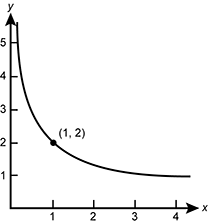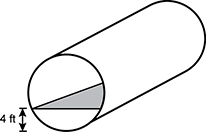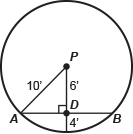# Study Guide

## Field 242: Multi-Subject: Secondary Teachers (Grade 7– to Grade 12) Part Two: Mathematics

### Sample Selected-Response Questions

The following reference material will be available to you during the test:

#### Competency 0001 Number and Quantity

1. Grapes are 80 percent water by weight and raisins are 15 percent water by weight. If 100 pounds of grapes are dried to make raisins, which weight is closest to the weight of the raisins?

1. 24 pounds
2. 32 pounds
3. 75 pounds
4. 85 pounds
Correct Response: A. This question requires the examinee to use proportional relationships to solve a multistep ratio and percent problem. If grapes are 80 percent water by weight, then 20 percent of the weight is not water, so 100 pounds of grapes contain 20 pounds of non-water matter. These 20 pounds must make up the non-water matter in the raisins. Since raisins are 15 percent water by weight, the non-water matter is 85 percent of the weight, so 20 equals 0.85 (total weight) and total weight of the raisins is 20 divided by 0.85 or about 24 pounds.

#### Competency 0002 Algebra and Functions

2. Use the graph below to answer the question that follows.Graph. Curved line starting high near the y axis and decreasing very quickly almost vertically then decreasing more slowly passing through the single point open parenthesis one comma two close parenthesis then slowing the decrease to an almost horizontal decrease.

The graph shows a function of the form  y equals f left paren x right paren equals c over square root of x  for constant c and  x is greater than 0  What is the inverse function of  y equals f left paren x right paren  for x is greater than zero?

1.  y equals two times square root of x
2.  y = 2 x squared
3.  y equals four over x squared
4.  y equals negative four over square root of x
Correct Response: C. This question requires the examinee to build new functions from existing functions. Since the graph contains the point (1, 2), substituting 1 for x and 2 for y calculates  c equals 2  Then  y equals two over square root of x  Inverse functions reverse the roles of x and y, so an equation for the inverse is  x equals two over square root of y  Squaring both sides,  x squared equals four over y    which yields   x squared y equals 4   which yields   y equals four over x squared

#### Competency 0003 Geometry and Statistics

3. Use the diagram below to answer the question that follows.right cylinder with a rectangular cross section length wise inserted inside of it that is four feet up from the bottom.

The fuselage of an airplane is modeled as a right circular cylinder with a diameter of 20 feet and a length of 200 feet. A platform is built into the cylinder to serve as a floor for cargo, as shown in the cross-section diagram. How much floor space, in square feet, is provided if the platform is positioned 4 feet above the bottom of the fuselage?

1. 2400 feet squared
2. 3200 feet squared
3. 4800 feet squared
4. 9600 feet squared